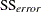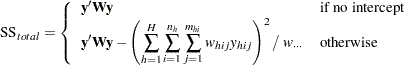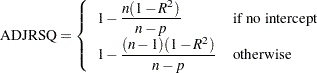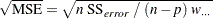Computational Details

Analysis of Variance (ANOVA)

PROC SURVEYREG  produces an analysis of variance table for the model specified in the MODEL statement. This table is identical to the one produced by the GLM procedure for the model. PROC SURVEYREG  computes ANOVA table entries by using the sampling weights, but not the sample design information about stratification and clustering.

The degrees of freedom (df) displayed in the ANOVA table are the same as those in the ANOVA table produced by PROC GLM. The Total DF is the total degrees of freedom used to obtain the regression coefficient estimates. The Total DF equals the total number of observations minus 1 if the model includes an intercept. If the model does not include an intercept, the Total DF equals the total number of observations. The Model DF equals the degrees of freedom for the effects in the MODEL statement, not including the intercept. The Error DF equals the Total DF minus the Model DF.

Multiple R-Square

PROC SURVEYREG  computes a multiple R-square for the weighted regression aswhereis the error sum of squares in the ANOVA tableandis the total sum of squareswhereis the sum of the sampling weights over all observations.

If you specify the ADJRSQ option in the MODEL statement, PROC SURVEYREG  computes an multiple R-square adjusted as the weighted regression aswhereis the multiple R-square.

Root Mean Square Errors

PROC SURVEYREG  computes the square root of mean square errors aswhereis the sum of the sampling weights over all observations.